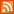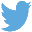Bucaro TecHelpHTTPS Encryption not required because no account numbers orpersonal information is ever requested or accepted by this siteCustom Search# How to Use Boolean Expressions in Java

By Doug Lowe

A boolean type can have one of two values: true or false. A boolean is used to perform logical operations, most commonly to determine whether some condition is true. For example:

boolean enrolled = true;
boolean credited = false;

Here, a variable named enrolled of type boolean is declared and initialized to a value of true, and another boolean named credited is declared and initialized to false.

WARNING! In some languages, such as C or C++, integer values can be treated as a boolean, with 0 equal to false and any other value equal to true. Not so in Java. In Java, you can't convert between an integer type and a boolean type.

A Boolean expression is a Java expression that, when evaluated, returns a Boolean value: true or false. Boolean expressions are used in conditional statements, such as if, while, and switch.

The most common Boolean expressions compare the value of a variable with the value of some other variable, a constant, or perhaps a simple arithmetic expression. This comparison uses one of the following relational operators:

 Operator Description == Returns true if the expression on the left evaluates to the same value as the expression on the right. != Returns true if the expression on the left does not evaluate to the same value as the expression on the right. < Returns true if the expression on the left evaluates to a value that is less than the value of the expression on the right. <= Returns true if the expression on the left evaluates to a value that is less than or equal to the expression on the right. > Returns true if the expression on the left evaluates to a value that is greater than the value of the expression on the right. >= Returns true if the expression on the left evaluates to a value that is greater than or equal to the expression on the right.

A basic Boolean expression has this form:

expression relational-operator expression

Java evaluates a Boolean expression by first evaluating the expression on the left, then evaluating the expression on the right, and finally applying the relational operator to determine whether the entire expression evaluates to true or false.

For example, suppose you have declared two variables:

int i = 5;
int j = 10;

Here are a few simple expressions along with their results:

 Expression Value Explanation i == 5 true The value of i is 5. i == 10 false The value of i is not 10. i == j false i is 5, and j is 10, so they are not equal. i == j – 5 true i is 5, and j – 5 is 5. i > 1 true i is 5, which is greater than 1. j == i * 2 true j is 10, and i is 5, so i * 2 is also 10.

WARNING! The relational operator that tests for equality is two equal signs in a row (==). A single equal sign is the assignment operator. When you're first learning Java, you may find yourself typing the assignment operator when you mean the equal operator, like this:

if (i = 5)

Oops. That's not allowed.

Do not test strings by using relational operators, including the equal operator. The correct way to compare strings in Java is to use the String.equals method.

You can combine two or more relational expressions in a single Boolean expression by using logical operators.

This is an excerpt from:.menubtn { margin-top:10px; margin-left:50px; width:220px; opacity:1; border-style:solid; border-color:#009000; background-color:#66ff66; transition-property: opacity; transition-duration: 1s; transition-timing-function: ease-out; } .menubtn:hover { opacity:1; background-color:#e5ff23; } Menu - More Java Programming MenuRSS FeedFollow @Stephen Bucaro Java